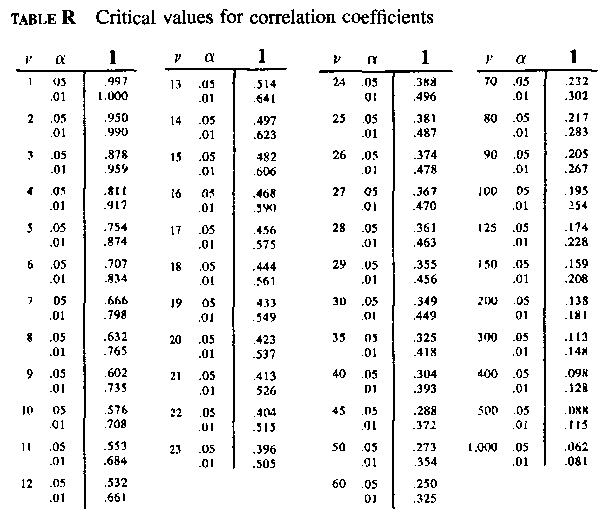Critical Values for the correlation coefficient r

Consult the table for the critical value of v = (n - 2) degrees of freedom, where n = number of paired observations. For example, with n = 28, v = 28 - 2 = 26, and the critical value is 0.374 at a =  0.05 significance level. Thus, an observed r = 0.31 in a sample of n=28 observations is not significantly different from random.

Table © 1995 by Rohlf & Sokal